## Title: plot-barplot-1
## Author: Paul Johnson <pauljohn at ku.edu>
## Date posted: 2013-02-05
## Description. Basic exploration of barplot

## One big hassle is that R barplot() assumes we
## provide the table of bar heights.  We get one
## "set" of bars for each row in the table.

## Start easy:

## Suppose we know how high we want the bars.
##

myBars <- c(0.2, 0.4, 0.5)
barplot(height = myBars)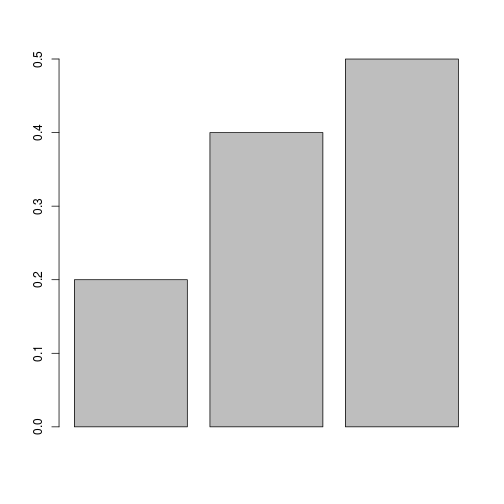## fiddle the width of the bars
barplot(height = myBars, width = c(0.2, 1, 0.3))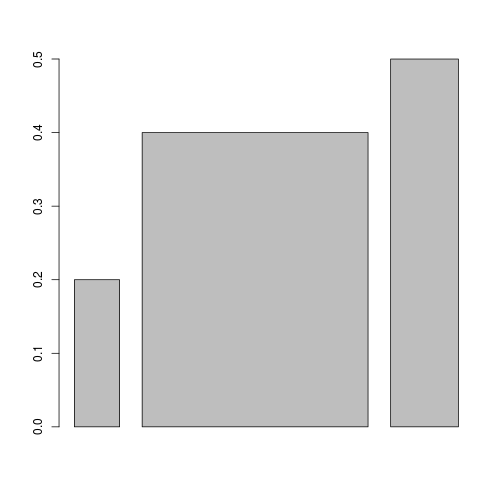## Sometimes we have 2 rows of data to plot. That
## is a matrix

myBars <- matrix(c(0.2, 0.4, 0.5, 0.33, 0.7, 0.1), nrow = 2, byrow = TRUE)

barplot(height = myBars)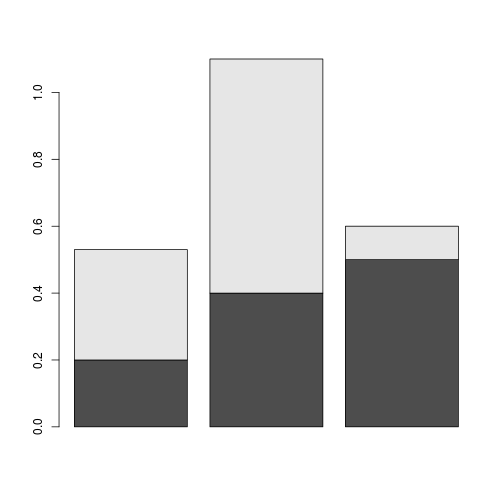## I rather have side-by-side plot
barplot(height = myBars, beside = TRUE)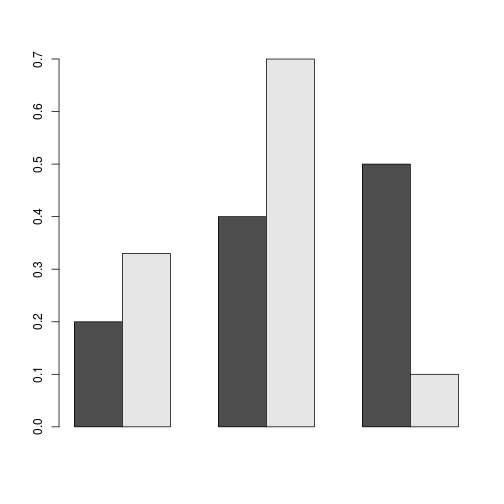## We will play games with barplot, but first
## "HOW DO WE GET THE NUMBERS WE WANT TO PLOT?"

## Well, it depends on what you are trying to show.

## Answer: I usually use a 2 step process.
## Step 1. table() gets the counts
## Step 2. prop.table() converts that to proportions.

## Let's make up some categorical variables for testing

## Here is 100 scores for x1
set.seed(234234)
x1 <- sample(c("Red","Black","Blue"), size = 100, replace = TRUE)
x2 <- sample(c("male","female"), size = 100, replace = TRUE)

x1 <- as.factor(x1)
x2 <- as.factor(x2)

plot(x2, x1)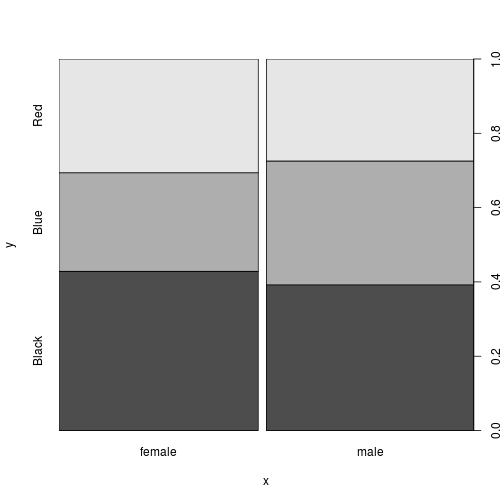## I want a side-by-side barplot instead.

## So we have to manufacture the data ourselves
table(x2, x1)

##         x1
## x2       Black Blue Red
##   female    21   13  15
##   male      20   17  14

t1 <- table(x2, x1)
t1.prop <- prop.table(t1, margin = 2)

## See: column proportions.
t1.prop

##         x1
## x2        Black   Blue    Red
##   female 0.5122 0.4333 0.5172
##   male   0.4878 0.5667 0.4828

barplot(height = t1.prop, beside = TRUE)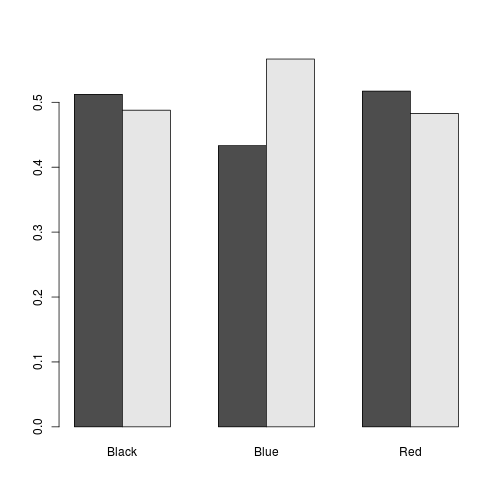## Sometimes you want the average value of a variable
## to serve as the height of the bars.  R leaves us (too) many
## different ways to get that. I like "aggregate".

## Lets make up a numeric variable

x3 <- rnorm(100, m = 40, s = 20)

## We need the result to be a table, with one column for male, one
## for female, and rows for Red Black Blue

## My first instinct was to calculate like this
aggregate(x3, by = list(x1,x2), mean)

##   Group.1 Group.2     x
## 1   Black  female 37.00
## 2    Blue  female 44.43
## 3     Red  female 45.39
## 4   Black    male 44.82
## 5    Blue    male 50.72
## 6     Red    male 43.63

## But the data is not formatted in a way that easily
## goes into a table.

## The gdata package has a nice function that does it
## called aggregate.table, but if you run it like so:
## library(gdata)
## aggregate.table(x3, by1 = x1, by2 = x2, mean)
##
## it says the function is deprecated, and instead it
## suggests:

tapply(X = x3, INDEX = list(x1, x2), FUN = mean)

##       female  male
## Black  37.00 44.82
## Blue   44.43 50.72
## Red    45.39 43.63

## That looks ok

myBars <- tapply(X = x3, INDEX = list(x1, x2), FUN = mean)
barplot(height = myBars)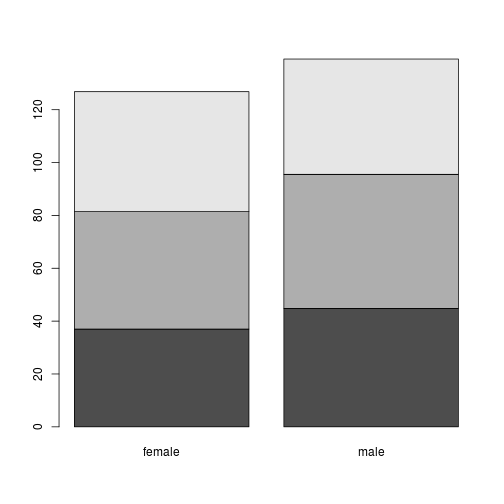barplot(height = myBars, beside = TRUE)

## Lets fiddle with the bar labels
##
barplot(height = myBars, beside = TRUE, names.arg = colnames(myBars))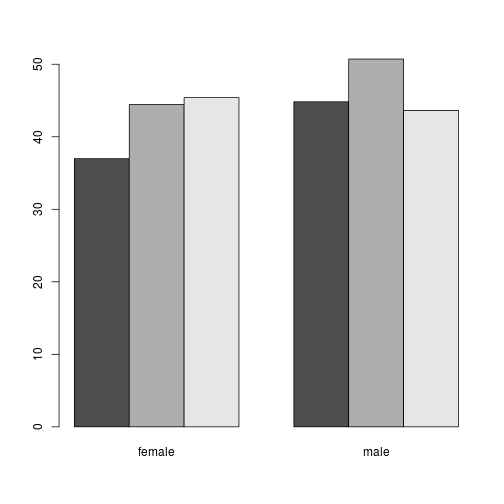##
barplot(height = myBars, beside = TRUE, names.arg = c(row.names(myBars),row.names(myBars)))

## I'd like to get both labels.
## Unfortunately, if we want to add labels for the groups.

## But we don't know where to place the labels. For example
mtext(colnames(myBars), side = 1, line = 2, at = c(1,2))
## This is no good as well.
mtext(colnames(myBars), side = 1, line = 2, at = c(4,8))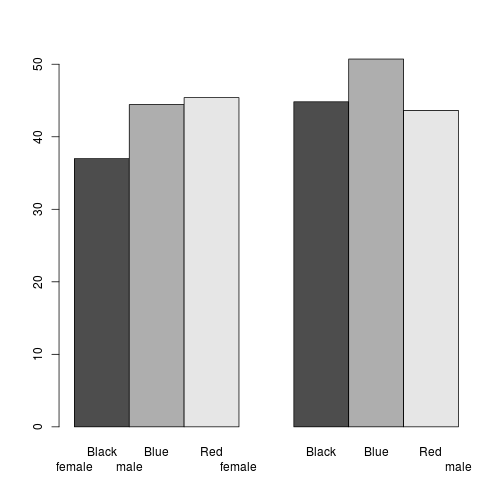## So, we have to ask the barplot for its coordinate system,
## like this:

bp1 <- barplot(height = myBars, beside = TRUE, names.arg = c(row.names(myBars),row.names(myBars)))

## Inspect bp1, it is just positions on the horizontal scale

bp1

##      [,1] [,2]
## [1,]  1.5  5.5
## [2,]  2.5  6.5
## [3,]  3.5  7.5

## The positions of the bars are there, but you have to look at the layout
## for a while to make sense of it.

## The numbers indicate that the "center" for each group of bars is found at
## positions 2.5 and 6.5.

mtext(colnames(myBars), side = 1, line = 3, at = c(2.5, 6.5))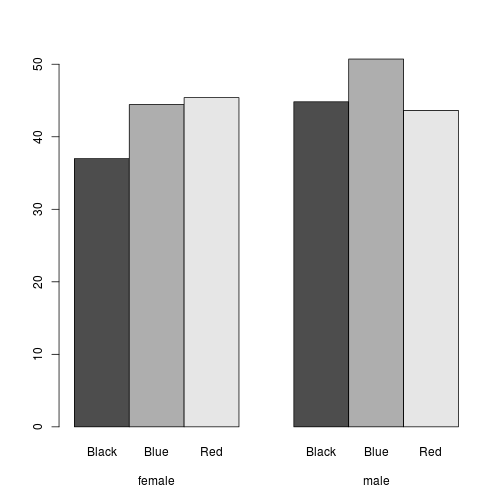## The end
##
##

##
##
## But wait, there's more.  Apparently I wrote the exact same
## example before. And forgot. How silly.

## Anyway, in case more examples help.

## Paul Johnson
## barplot data input. What a Hassle.
## 2011-06-22

## Here's a case where it is best to understand what R wants,
## before trying to work your example.  Or end goal is to
## make a grouped barplot.

## Start easy, give barplot one column

x <- c(.14, .23, .66)
barplot(x)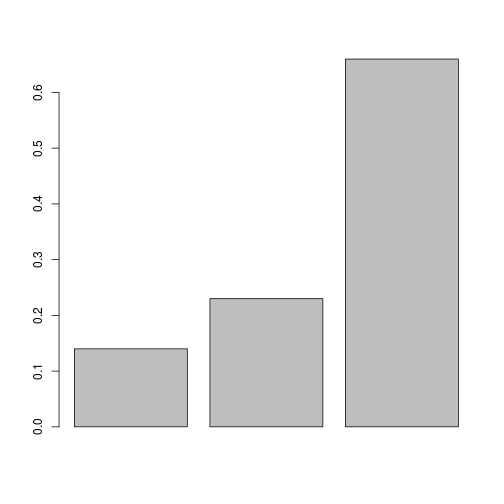## table or aggregate can produce same kind of thing
## Get some small integers in a data frame
rawdata <- rpois(200, lambda = 2)

x <- table(rawdata)

## Convert to proportions

x <- x / sum(x)

barplot(x)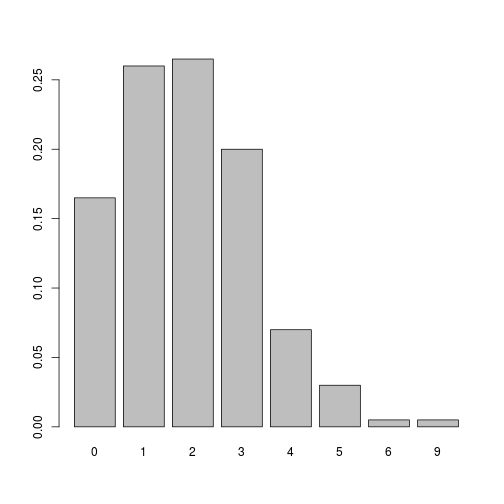## Now work on richer information

## Suppose the input is a matrix with 2 columns

x <- matrix( c(.14, .23, .66, .44, .53, .55), ncol = 2)

## look at x

x

##      [,1] [,2]
## [1,] 0.14 0.44
## [2,] 0.23 0.53
## [3,] 0.66 0.55

barplot(x)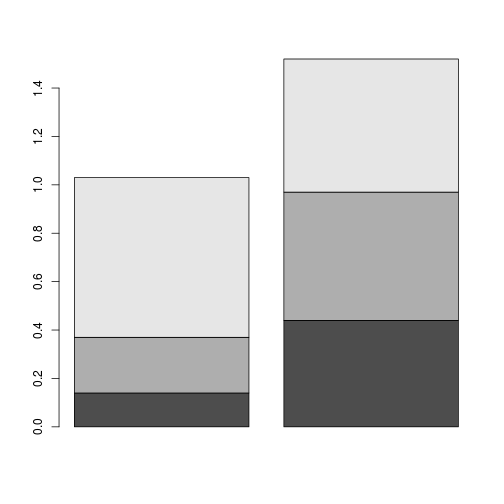## I hate stacked charts

## I think this would be called a grouped bar plot.
barplot(x, beside = TRUE)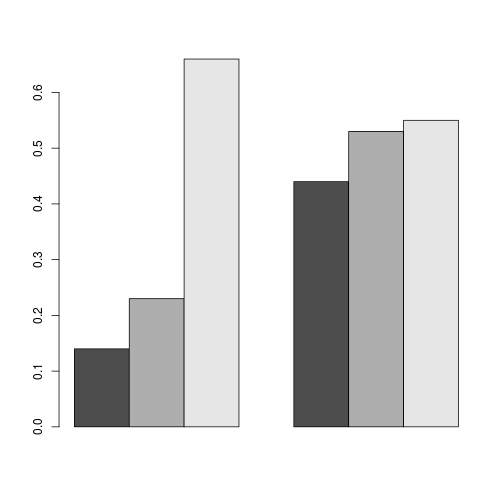## That has no names because my input table had no names.

## Think of the columns as sex
colnames(x) <- c("Male","Female")
x

##      Male Female
## [1,] 0.14   0.44
## [2,] 0.23   0.53
## [3,] 0.66   0.55

## Row represents cities
rownames(x) <- c("NY","LA","SF")
x

##    Male Female
## NY 0.14   0.44
## LA 0.23   0.53
## SF 0.66   0.55

barplot(x, beside = TRUE)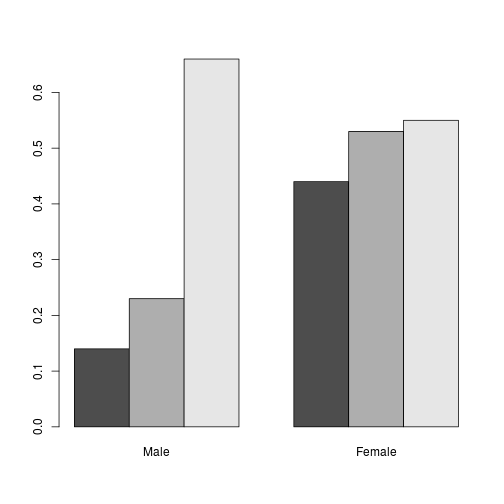## How to decorate that?
## Name individual bars? OK:
barplot(x, beside = TRUE, names.arg = c("A","B","C","D","E","F"))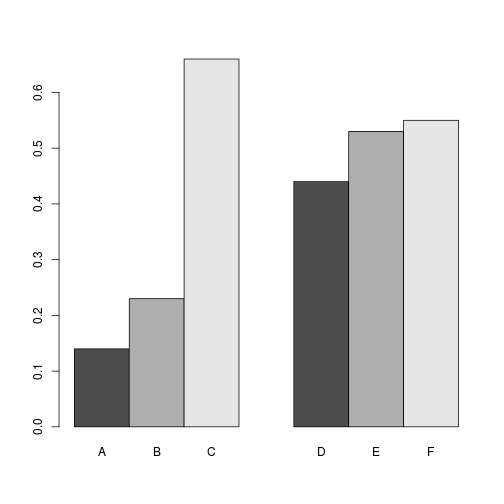## Instead, lets go for two-layered output.
## Grab the output from barplot in order
## to see where bars are positioned.
bp1 <- barplot(x, beside = TRUE)

mtext(text = c("first","second","third"), side = 1, line = 0, at= bp1[,1])

mtext(text = c("fourth","fifth","sixth"), side = 1, line = 0, at = bp1[ ,2])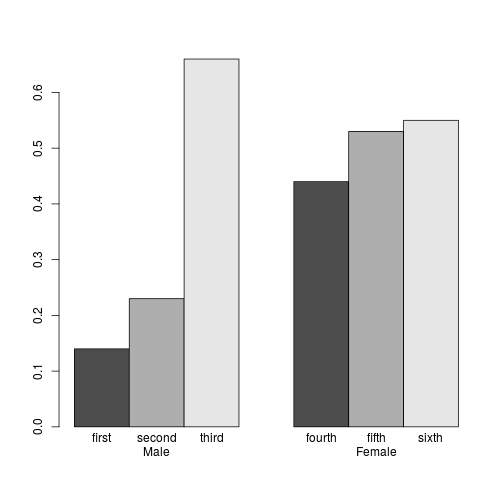## Instead, lets write vertically inside the bars!
## Let's write at one-half of the column's height (that's why
## I have 0.5*x in the text commands

bp1 <- barplot(x, beside = TRUE)
text( bp1[ ,1], 0.5*x[ ,1], c("first","second","third"))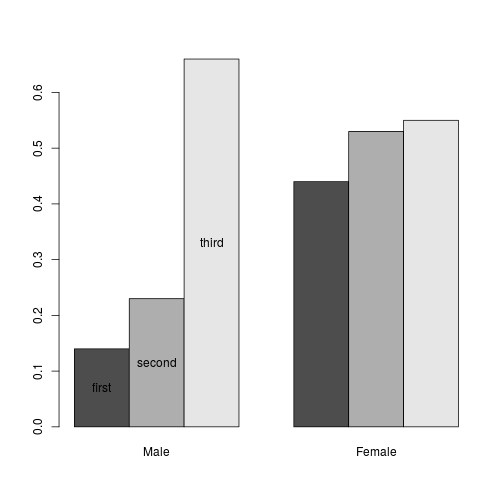## srt will rotate text strings by degree
bp1 <- barplot(x, beside = TRUE)
text( bp1[ ,1], 0.5*x[ ,1], c("first","second","third"), srt = 66)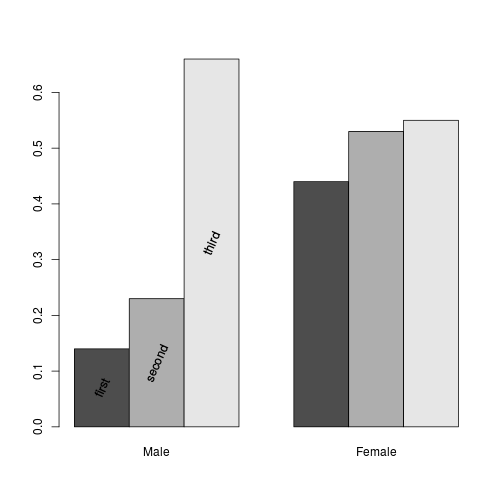bp1 <- barplot(x, beside = TRUE)
text( bp1[ ,1], 0.5*x[ ,1], c("first","second","third"), srt = 90)
text( bp1[ ,2], 0.5*x[ ,2], c("first","second","third"), srt = 90)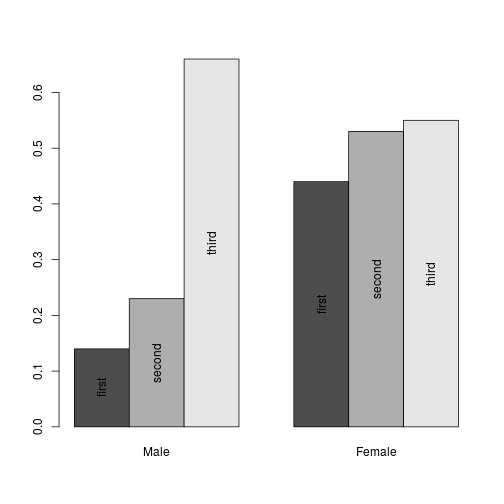### Note problem: Fill colors in legend not correct
bp1 <- barplot(x, beside = TRUE)
legend("topleft", legend = c("first","second","third"), fill = c(1,2,3))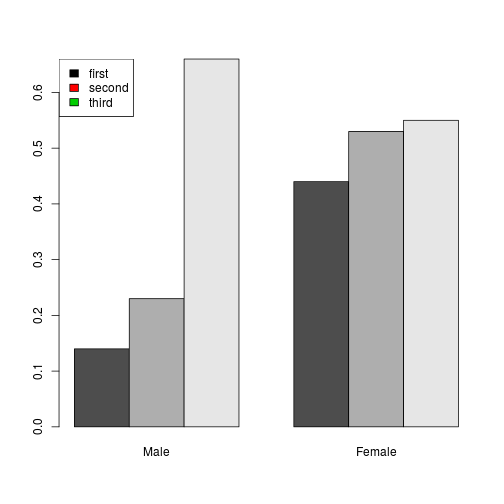### Need to figure out what colors barplot uses
### I believe it is drawing colors from the function "gray.colors"
gray.colors(3)

##  "#4D4D4D" "#AEAEAE" "#E6E6E6"

bp1 <- barplot(x, beside = TRUE)
legend("topleft", legend = c("first","second","third"), fill= gray.colors(3))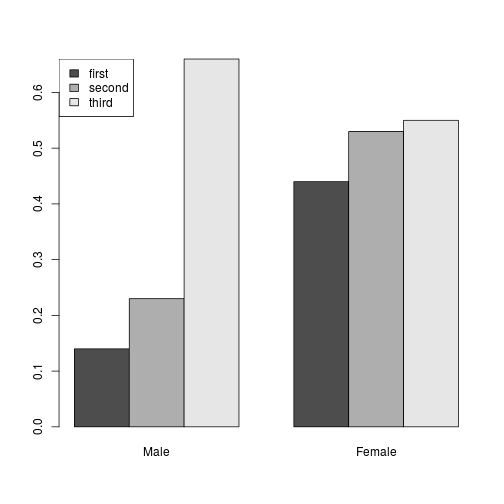### So, what do you get out of this?

### barplot wants you to give it a matrix, one column per group of bars.

### So if your data is like this

### data
### id  sex region iq age
### 01  M    W     122 12
### 02  F    W     111 08
### 03  M    E     89  07
### 04  F    S     144 19
### 05  F    N     123 44

### You want a barplot that shows this the
## mean "iq" subdivided by sex, then region

##          | |               |
##        | | |               |
##      | | | |               | | | |
##      E W N S               E W N S

##         Male                 Female

## So we need a matrix with 2 columns, Male and Female,
## Rows for regions and cells are means.

## First, manufacture the data

id <- 1:1000
sex <- sample(x= c("M","F"), size = 1000, replace = T)
region <- sample(x= c("E","W","N","S"), size = 1000, replace = T)
iq <- rnorm(1000, m = 100, sd = 15)
age <- rpois(1000, lambda = 20)
dat <- data.frame(id, sex, region, iq, age)

## Use R's "aggregate" go produce that
aggdat <- aggregate(dat\$iq, by = list(sex = sex,region = region), FUN= mean)
aggdat

##   sex region      x
## 1   F      E  99.36
## 2   M      E 100.40
## 3   F      N 101.35
## 4   M      N 100.23
## 5   F      S  96.31
## 6   M      S 100.23
## 7   F      W  99.07
## 8   M      W  99.13

colnames(aggdat) = "meaniq"

## aggdat is in the "long" format, but we need the "wide" format
x <- unstack(aggdat, meaniq ~ sex )
x <- as.matrix(x)
barplot(x, beside = TRUE)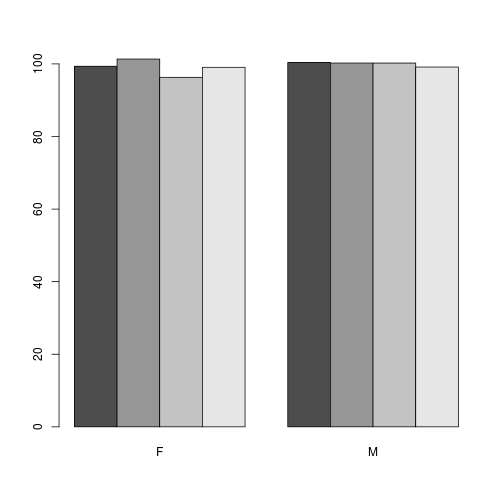## And I think that's all I need to show